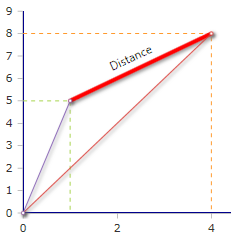# Distance Function

Calculates the distance between two points

### Description

The function $$Distance$$ calculates the distance between two points in the coordinate system. The endpoints of the distance are defined with their X / Y coordinates or two objects of class $$Point$$.

### Syntax

Distance (x1, y1, x2, y2)

Distance (Point(x, y), Point(x, y))

### Example

Distance(Point(1,5),Point(4,8))= 4.24### Use of class Point

By using the $$Point$$ objects, the distance can also be calculated with arguments other than $$x / y$$. The class $$Point$$ contains its own methods for calculating the properties.

### Example

Suppose only the x-coordinates are known. $$y$$ is unknown, but the angles from the 0 point can be determined

For Point a we determine an angle to the x-axis of 78.69° and for Point b 63.43°

In the initialization of $$Point$$ a command string must be inserted to tell that the arguments are the position $$x$$ and the angle $$alpha$$.

Distance(Point("x,α",1,78.69),Point("x,α",4,63.43))= 4.24